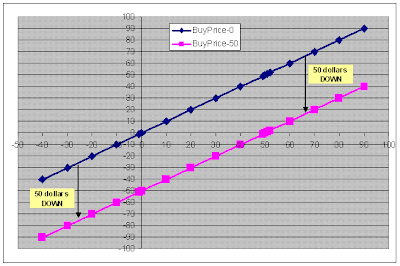Option Trading Tutorial Part 5: Payoff Functions Summary: Options, Futures, Derivatives & Commodity Trading

# Option Trading Tutorial Part 5: Payoff Functions Summary

Continuing further from the 2nd part Price Adjustments in Payoff Functions, here is the 5th part of the series on option trading tutorials.Now, the tutorial for this part begins here: Please read the following important things:

Point no. 1:

The first thing that you should note is that the BLUE line is for ZERO Buy price, while the PINK Line is for the 50 Dollars buy price.
What we observe is that as compared to Zero buy price graph (BLUE), the 50 dollar buy price graph (PINK) is 50 units below the zero priced graph.
Hence, the first lesson that you should note is

Whenever there is a price to be paid for certain stock, option or commodity, we should begin by drawing a ZERO priced graph and then shift the zero priced graph down by the amount of actual price paid (50 dollars in this case)

Hence, in the above case, we started with a zero priced BLUE graph assuming that the stock was available for free at Zero dollars. And when we became more realistic and introduced the price, we shift the zero priced graph down by 50 dollars to get the actual stock position, which is the PINK graph.

Point no. 2:

The second thing to note is that the graphs clearly tell us the RISK and the RETURN areas.

The RETURN area is the area where the graph stays ABOVE the horizontal axis or the x-axis, while the RISK area is the region which stays BELOW the horizontal axis or the x-axis. Return area is also categorized by a PROFIT area and RISK area is categorized as LOSS making region.

Have a look at the following figure describing Risk and return areas for the 2 BLUE and PINK graphs:IN the above figure, the RISK areas are plotted with the DOWN arrows, while the return areas are plotted with the UP arrows.

For the zero priced BLUE graph, the risk area starts as soon as the stock price falls below ZERO (or the BUY price). Similarly, for the 50 dollar priced PINK graph, the risk are starts as soon as the stock price falls below 50 dollars (or the Buy price).

On the other hand, the Return for the Blue graph starts as soon as the stock price goes above Zero (or the Buy price), while for the Pink graph, the return area starts as soon as the stock price goes above 50 dollars (or the buy price).

So in summary, today we have learnt 2 important things:
1) For any investment, start with a Zero price graph and then shift it down with the amount of price that you pay for the investment.
2) Clearly identify the Risk and Return areas (also known as Loss and Profit areas), as the areas where the graph crosses the horizontal axis (or x-axis).

Now that you are familiar with the basic working of the options (calls and puts), its time to check the trading basics of some real options. Here are the next steps (advised to go in the order listed):

Lets now head on to the next part - the real payoff functions for derivatives: Payoff Functions for Options & Futures: Explained with Examples
 Posted by Shobhit See All Articles with